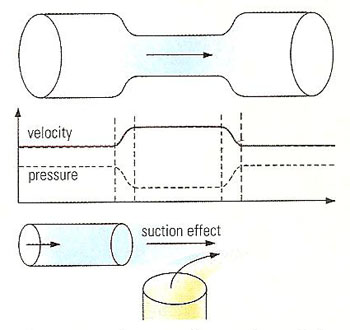A# Bernoulli's lawBernoulli's law is a statement of the conservation of energy in a form useful for solving problems involving fluids. For a non-viscous, incompressible fluid in steady flow, the sum of pressure, and potential and kinetic energies per unit volume is constant at any point. That is:

p + ½ρV 2 + ρgh = constant

where p is the pressure, ρ the density, V the velocity, and h the height in a gravitational field of strength g, all measured at the same point. This quantity is then constant throughout the fluid.

Using this relationship, which was formulated by Daniel Bernoulli, it is possible to measure the velocity of a fluid by measuring its pressure at two points, as with a manometer or a pitot tube.

Bernoulli's principle is used for, but not essential to, lift by airplanes. Another situation in which it applies is cavitation.